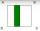Equilateral triangle v2

Equilateral triangle has a perimeter 36 dm. What is its area?

Result

S =  62.354 dm2

Solution:Try calculation via our triangle calculator.

Leave us a comment of example and its solution (i.e. if it is still somewhat unclear...):Be the first to comment!To solve this example are needed these knowledge from mathematics:

See also our trigonometric triangle calculator. Pythagorean theorem is the base for the right triangle calculator.

Next similar examples:

1. ISO Triangle V2Perimeter of RR triangle (isosceles) is 474 m and the base is 48 m longer than the arms. Calculate the area of this triangle.
2. Isosceles IIIThe base of the isosceles triangle is 17 cm area 416 cm2. Calculate the perimeter of this triangle.
3. Triangle SAAThe triangle has one side long 71 m and its two internal angles is 60°. Calculate the perimeter and area of the triangle.
4. TriangleCalculate heights of the triangle ABC if sides of the triangle are a=75, b=84 and c=33.
5. Triangle - is RT?Triangle has a circumference of 90 cm. Side b is 1 cm longer than c, side c is 31 cm longer than side a. Calculate the length of sides and determine whether triangle is a right triangle.
6. Three sidesSide b is 2 cm longer than side c, side a is 9 cm shorter than side b. The triangle circumference is 40 cm. Find the length of sides a, b, c . .. .The double ladder shoulders should be 3 meters long. What height will the upper top of the ladder reach if the lower ends are 1.8 meters apart?
8. The farmerThe farmer would like to first seed his small field. The required amount depends on the seed area. Field has a triangular shape. The farmer had fenced field, so he knows the lengths of the sides: 119, 111 and 90 meters. Find a suitable way to determine thThe double ladder is 8.5m long. It is built so that its lower ends are 3.5 meters apart. How high does the upper end of the ladder reach?
10. Center traverseIt is true that the middle traverse bisects the triangle?
11. SinesIn ▵ ABC, if sin(α)=0.5 and sin(β)=0.6 calculate sin(γ)
12. Folded squareABCD is a square. The square is folded on the midpoint of AB and A is folded onto the fold, creating a shaded region. The perimiter of the shaded figure is 75. Find the area of square ABCD
13. SurveyorCalculate the area of ​​what may vary rectangular, if it focused by surveyor and found the dimensions 18 x 15 m while in each of the four joint points can be position deviation 25 cm?
14. Theorem proveWe want to prove the sentence: If the natural number n is divisible by six, then n is divisible by three. From what assumption we started?
15. Circular lawnAround a circular lawn area is 2 m wide sidewalk. The outer edge of the sidewalk is curb whose width is 2 m. Curbstone and the inner side of the sidewalk together form a concentric circles. Calculate the area of the circular lawn and the result round to 1
16. Linear systemSolve a set of two equations of two unknowns: 1.5x+1.2y=0.6 0.8x-0.2y=2
17. Nineteenth memberFind the nineteenth member of the arithmetic sequence: a1=33 d=5 find a19